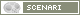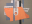OpenMusic DocumentationOM 6.6 User Manual > Basic Tools > Curves and Functions > BPF / BPC Tools
page précédentepage suivante

BPF and BPC Tools

This section details a set of important concept and tools. Among these, a number of function boxes allow to build or manipulate BPFs and BPCs. To access these tools, go to Functions / Basic Tools / Curves & Functions.

Online Documentation Reference

The online function reference provides more detailed information about the following (and more) functions, as well as different arguments. To refer to it, select a function and press d.

Getting Coordinates : Transfer and Point Coordinates

Point-Pairs : Points of the Curve

The point-pairs function returns the points of the curve as a list of (x y) coordinates.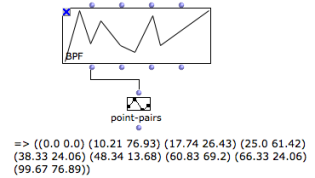Point-pairs : list of (x y) points in the curve.
Transfer : Selected Points

Two transfer tools return the values of points that are not explicitly given in the curve.

• "X-transfer" returns the ordinates for a given abscissa.

• "Y-transfer" returns the abscissa values corresponding to given ordinates.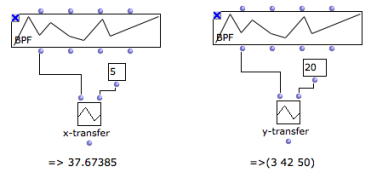Transfers : x-transfer returns the y-coordinate for x=5. Y-transfer returns the various abscissa for y=20.

Sampling a Curve : Om-Sample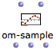Sampling consists in creating a new curve of n regularly sampled points, out of an initial set of points.
Inputs
 The om-sample function has two default inputs : "self" : a list of points, BPF or BPC objects. "sample-rate" : a number of samples in integers, or a sample-rate in floats. It has three optional inputs :"x-min" and "x-max" define a segment to sample within the curve."decimals" :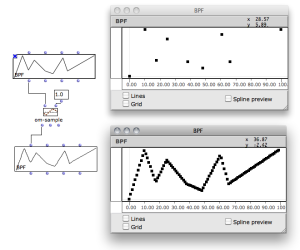The sampling rate can be expressed as a float input to Om-samplezoom
"sample-rate" input : Integer or Float

Be a curve whose min = 0 and max = 100.

• Integer : n = 20 we get 20 points, whose values are (0 5 10 15 20 25 30 35 .... 100).
• Float : SR = 20.0, we get 5 points, whose values are (0 20.0 40.0 60.0 80.0 100.0).

In any case n = (xmax - xmin) / SR

Outputs

Om-sample returns 3 values :

• a sampled object, such as a BPF , BPC ...
• a list of x -coordinates
• a list of y -coordinates
Up-Sampling and Down-Sampling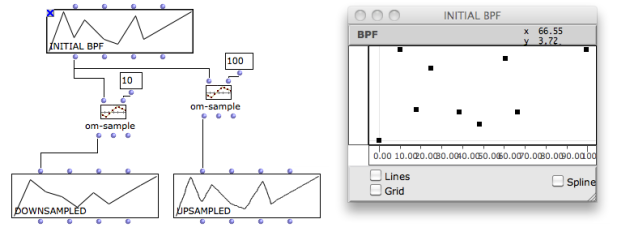If n is lower than the initial number of points, curves are down-sampled. If n is higher, curves are up-sampled.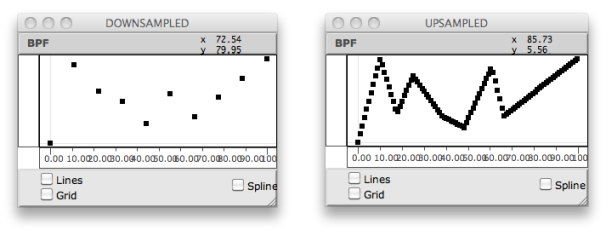Splines : Om-Spline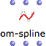Splines are mathematical functions defined piecewise by polynomial interpolation. They are used for generating smoothed interpolated shapes , starting from a set of control points. The om-spline function generates spline curves starting from the points of a BPF or BPC.
Inputs
• "resolution" : a number of points in the spline preview.

• "degree" : a number of adjacent control points used for computing the spline curve points. Higher values produce smoother curves.

Outputs
 The three outputs of om-spline return :a spline object (BPF, BPC...)a list of x-coordinatesa list of y-coordinates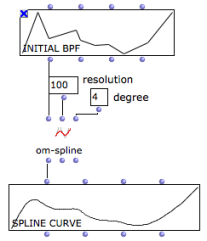Spline Preview

The BPF and BPC editors allow to visualize a preview of the output spline object.

Spline Preview in the BPF/BPC Editors.
page précédentepage suivante
A propos...(c) Ircam - Centre Pompidou